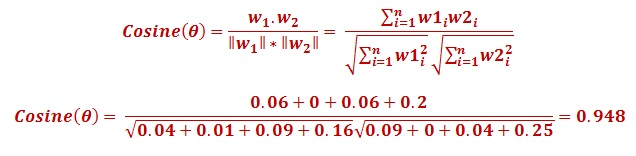Advanced Database Management System - Tutorials and Notes: Natural Language Processing MCQ 12

# MCQ in Natural Language Processing, Quiz questions with answers in NLP, Top interview questions in NLP with answers, language model quiz questions, MLE in NLP

## Multiple Choice Questions and Answers in NLP Set - 12

1. Assume a corpus with 350 tokens in it. We have 20 word types in that corpus (V = 20). The frequency (unigram count) of word types “short” and “fork” are 25 and 15 respectively. If we are using the Laplace smoothing, which of the following is PLaplace(“fork”)?

(a) 15/350

(b) 16/370

(c) 30/350

(d) 31/370

 Answer: (b) 16/370 In Laplace smoothing (also called as Add-1 smoothing), we find the probability by adding 1 with the numerator and V with the denominator. This is to ensure that frequency of each word of the corpus is added with 1. P(w) = [count(w)+1] / [count(tokens)+V] = 16/370

2. When training a language model, if we use an overly narrow corpus, the probabilities

(b) Reflect all possible wordings

(c) Reflect intuition

(d) Don’t generalize

 Answer: (d) Don’t generalize Due to the output of LMs being dependent on the training corpus, N-grams only work well for word prediction if the test corpus looks like the training corpus. Hence, if the training corpus is overly narrow corpus, the probabilities don’t generalize.

3. The difference(s) between generative models and discriminative models include(s)

(a) Discriminative models capture the joint distribution between features and class labels

(b) Generative models assume conditional independence among features

(c) Generative models can effectively explore unlabeled data

(d) Discriminative models provide more flexibility in introducing features.

 Answer: (c) and (d) Generative models can effectively explore unlabeled data. Discriminative models provide more flexibility in introducing features.

4. Assume that there are 10000 documents in a collection. Out of these, 50 documents contain the terms “difficult task”. If “difficult task” appears 3 times in a particular document, what is the TFIDF value of the terms for that document?

(a) 8.11

(b) 15.87

(c) 0

(d) 81.1

 Answer: (b) 15.9 IDF = log(total no. of docs/no. of docs with given terms) = log(10000/50) = 5.29 TFIDF = given term’s frequency in a doc * IDF = 3 * 5.29 = 15.87

5. Let us suppose that you have the following two 4-dimensional word vectors for two words w1 and w2 respectively:

w1 = (0.2, 0.1, 0.3, 0.4) and w2 = (0.3, 0, 0.2, 0.5)

What is the cosine similarity between w1 and w2?

(a) 0.948

(b) 0.832

(c) 0

(d) 0.5

 Answer: (a) 0.948 Cosine similarity can be calculated as follows;For the given problem, n=4. w1.w2 is the dot product which can be expanded for our data as follows; w1.w2 = (0.2 * 0.3) + (0.1 * 0) + (0.3 * 0.2) + (0.4 * 0.5)

*************